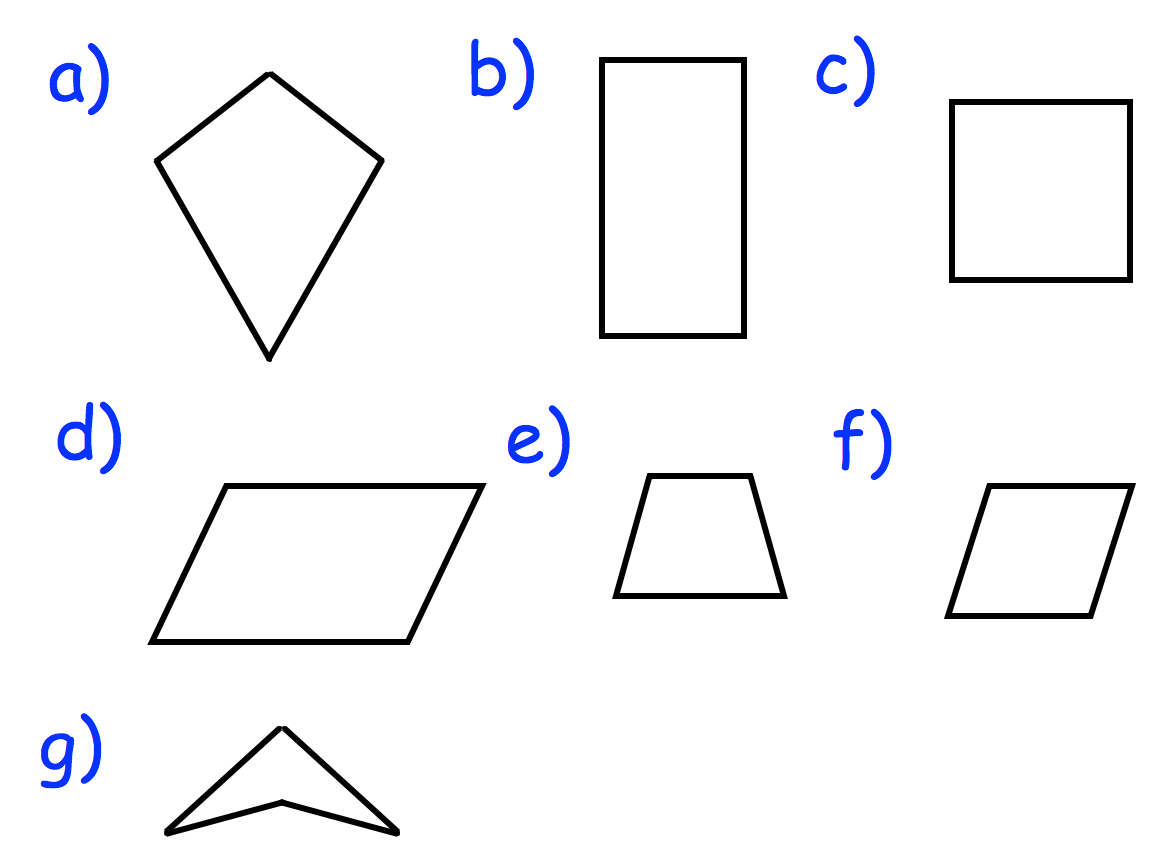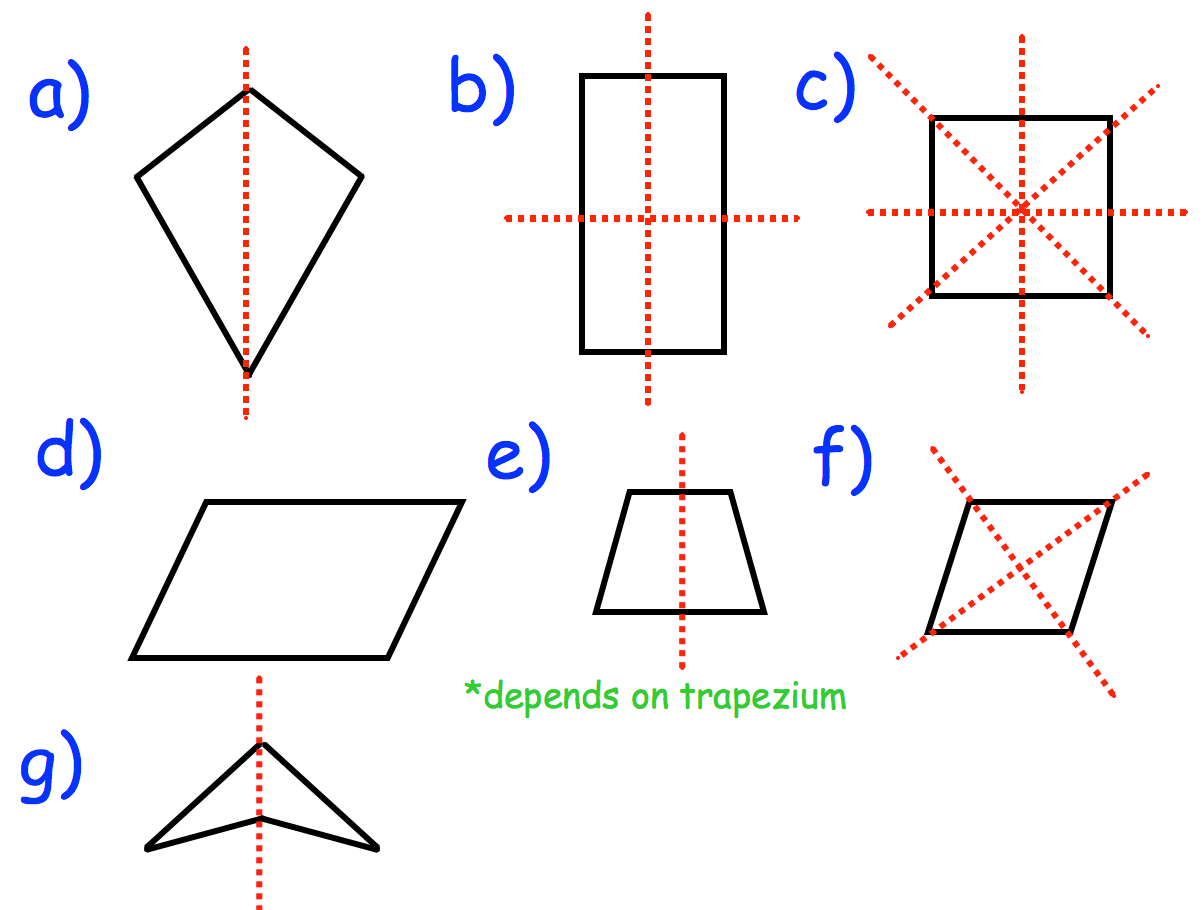July 16, 2016

Workout – Question 1Question 2

(a)  Kite          (b)  parallelogram          (c)  square          (d)   Trapezium

(e)  Rectangle         (f)   Rhombus

Question 3:Question 4:

(a)  Order 4          (b)   Order 2          (c)   Order 1          (d)   Order 2

(e)  Order 1          (f)  Order 2

Question 5:  Some trapeziums (trapezia)

Question 6:  Kites, Rectangles, Parallelograms, Arrowheads/Deltas

Question 7:  Squares and rhombuses (rhombi)

Question 8:  Squares, rectangles, Parallelograms, rhombuses (rhombi)

Question 9: Trapezium

Apply:

Question 1:  A trapezium may have no lines of symmetry, but it may also have one line of symmetry.

Question 2:

(a)  Correct – it does have four right angles

(b)  Incorrect – it has two pairs of parallel lines

(c)  Incorrect – a rectangle only has two lines of symmetry

(d)  Correct – it does have rotational symmetry of order 2.

Categories: Uncategorized